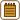## Thermal expansion of brass balls

A brass sphere has a diameter of 4 cm at 15 °C. How much do you need to increase its temperature in order for the sphere not to fit in a hole of 4.04 cm in diameter?

• #### Hint

To calculate the change in diameter with temperature, you need to know the so-called linear thermal expansion coefficient α, which you can find in the tables.

• #### Analysis

The sphere does not pass through the hole if its diameter increases to more than 4.04 cm. In order to determine the dependence of the diameter of the sphere on the temperature, we need to know the brass coefficient of linear thermal expansion α, which we find in the tables. We express the change of the temperature from the formula for thermal expansion and we calculate it using the appropriate values then.

• #### Notation

 t0 = 15 °C Initial temperature of the sphere d0 = 4 cm The initial sphere diameter d1 = 4.04 cm Hole diameter Δt = ? Temperature change

From the tables:

 α = 20·10−6 °C−1 Linear thermal expansion coefficient
• #### Solution

We have to determine at what temperature the diameter of the sphere will be equal to the diameter of the hole. In order to determine the dependence of the diameter on the temperature, we need to know the linear thermal expansion coefficient α (we are considering the change in one dimension, therefore we are working with the linear expansion coefficient).

$d_1=d_0\left(1+\alpha\mathrm{\Delta}t\right)$

We can express the desired temperature change Δt:

$\frac{d_1}{d_0}-1=\alpha\mathrm{\Delta}t$ $\mathrm{\Delta}t=\frac{1}{\alpha}\left(\frac{d_1}{d_0}-1\right)$

Numerical substitution:

$\mathrm{\Delta}t=\frac{1}{20\cdot{10^{-6}}}\left(\frac{4.04}{4}-1\right)\,{\,^{\circ}\mathrm{C}}=500{\,^{\circ}\mathrm{C}}$